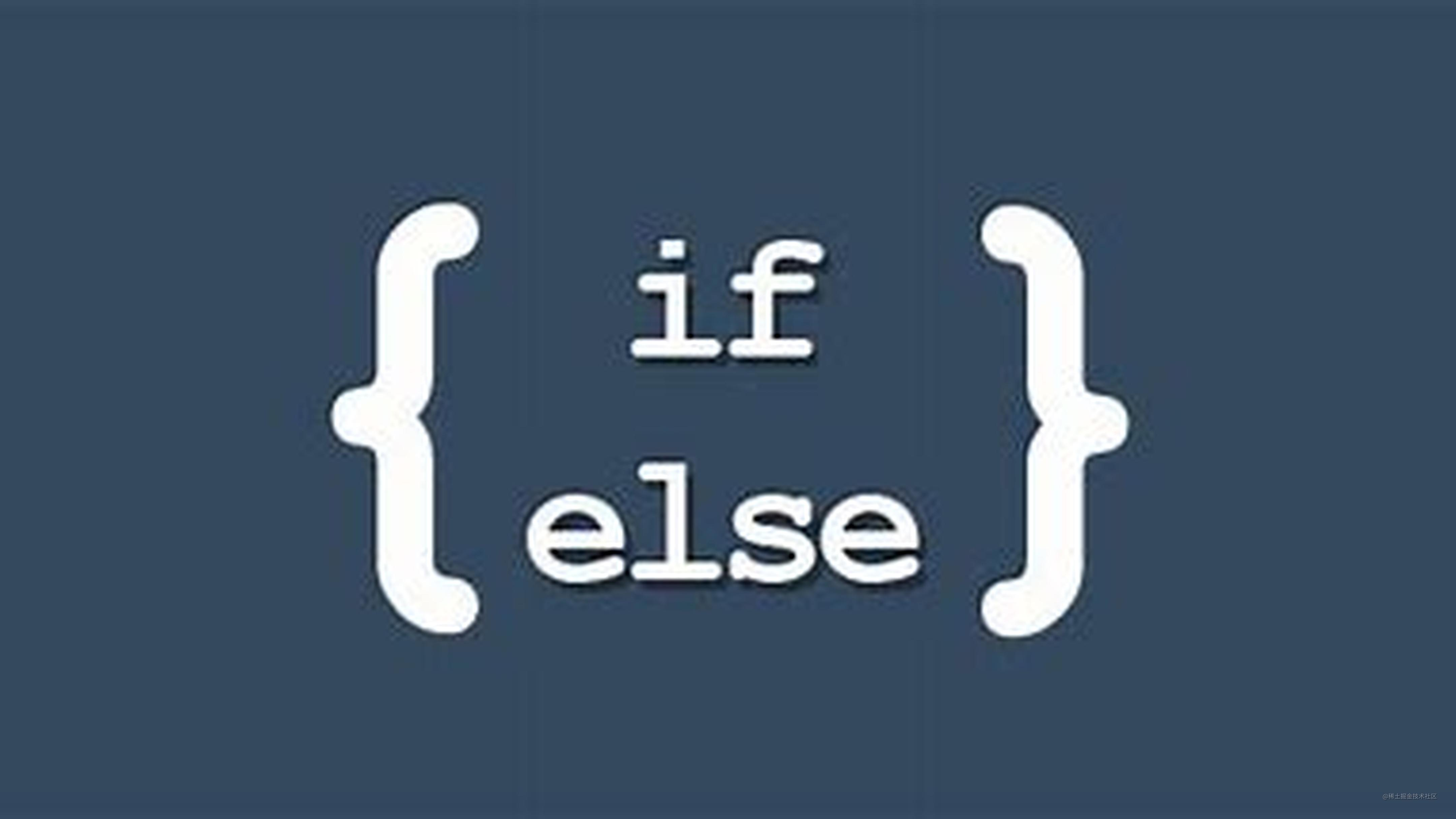# 提升代码可读性，减少if-else的几个小技巧“我正在参加「掘金·启航计划」”

## 短路运算💻

Javascript 的逻辑或 `||` 的短路运算有时候可以用来代替一些比较简单的 `if else`

• 逻辑或 `||` 的短路运算：若左边能转成true，返回左边式子的值，反之返回右边式子的值。

``````let c
if(a){
c = a
} else {
c = b
}

``````let c = a || b

## 三元运算符🎶

``````const fn = (nBoolean) {
if (nBoolean) {
return 1
} else {
return 0
}

}

// 使用三元运算符
const fn = (nBoolean) {
return nBoolean ? 1 : 0
}

``````// num值在nBoolean为true时为10，否则为5
let num = nBoolean ? 10 : 5

// 求0-n之间的整数的和
let sum = 0;
sum += n
return n >= 2 ? add(n - 1) : result;
};

## switch case🖥️

``````let type = 'A'

//if else if
if (type === 'A' || type === 'B') {
console.log(1);
} else if (type === 'C') {
console.log(2);
} else if(type === 'D') {
console.log(3);
} else {
console.log(0)
}

//switch case
switch (type) {
case 'A':
case 'B':
console.log(1)
break
case 'C':
console.log(2)
break
case 'D':
console.log(3);
break;
default:
console.log(0)
}

## 对象配置/策略模式📑

``````let type = 'A'

let tactics = {
'A': 1,
'B': 1,
'C': 2,
'D': 3,
default: 0
}
console.log(tactics[type]) // 1

### 案例1 商场促销价🙋

``````// 获取折扣 --- 使用if else
const getDiscount = (userKey) => {
if (userKey === '普通会员') {
return 0.9
} else if (userKey === '年费会员') {
return 0.85
} else if (userKey === '超级会员') {
return 0.8
} else {
return 1
}
}
console.log(getDiscount('普通会员')) // 0.9

``````// 获取折扣 -- 使用对象配置/策略模式
const getDiscount = (userKey) => {
// 我们可以根据用户类型来生成我们的折扣对象
let discounts = {
'普通会员': 0.9,
'年费会员': 0.85,
'超级会员': 0.8,
'default': 1
}
return discounts[userKey] || discounts['default']
}
console.log(getDiscount('普通会员')) // 0.9

``````// 获取折扣 -- 使用对象配置/策略模式
const getDiscount = (userKey) => {
// 我们可以根据用户类型来生成我们的折扣对象
let discounts = new Map([
['普通会员', 0.9],
['年费会员', 0.85],
['超级会员', 0.8],
['default', 1]
])
return discounts.get(userKey) || discounts.get('default')
}
console.log(getDiscount('普通会员')) // 0.9

### 案例2 年终奖🏆

``````const calculateBonus = (performanceLevel, salary) => {
if (performanceLevel === 'A'){
return salary * 4
}
if (performanceLevel === 'B'){
return salary * 3
}
if (performanceLevel === 'C'){
return salary * 2
}
}
calculateBonus( 'B', 20000 ) // 输出：60000

``````let strategies = new Map([
['A', 4],
['B', 3],
['C', 2]
])
const calculateBonus = (performanceLevel, salary) => {
return strategies.get(performanceLevel) * salary
}
calculateBonus( 'B', 20000 ) // 输出：60000

``````// 以绩效_部门的方式拼接键值存入
let strategies = new Map([
['A_D', 4 * 1.2],
['B_D', 3 * 1.2],
['C_D', 2 * 1.2],
['A_F', 4 * 0.9],
['B_F', 3 * 0.9],
['C_F', 2 * 0.9]
])
const calculateBonus = (performanceLevel, salary, department) => {
return strategies.get(`\${performanceLevel}_\${department}`) * salary
}
calculateBonus( 'B', 20000, 'D' ) // 输出：72000

## 结尾🔚

`if else`并没有歧视的意思，只是希望在大家以后的代码中不仅仅只有`if else`😨。